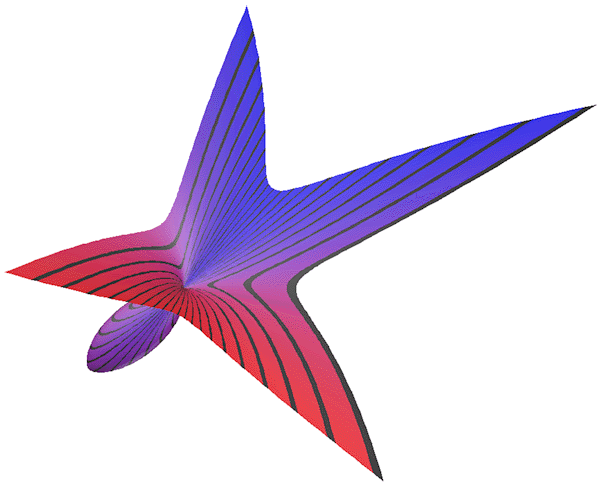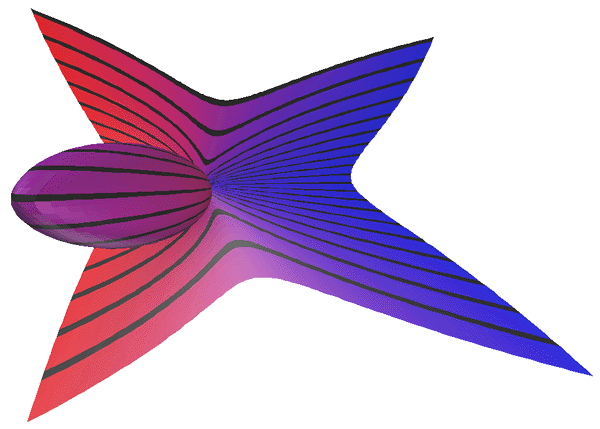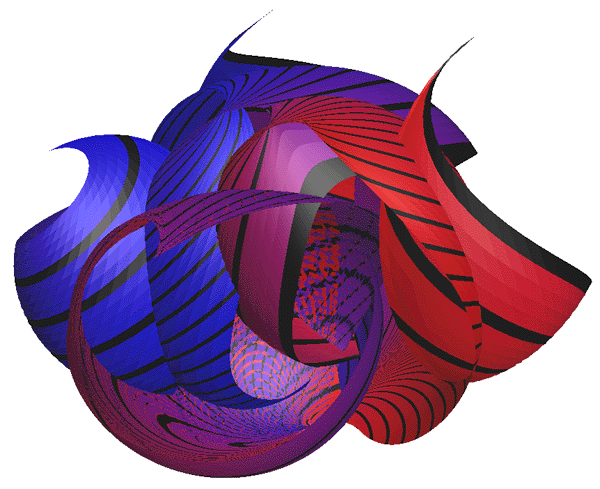# Twisted Plane

By Roger Bagula
Rendering by Paul Bourke
June 1999### C Source code by Paul Bourke

```#include "stdio.h"
#include "stdlib.h"
#include "math.h"
#include "paulslib.h"

int N = 100;
double umin = -1, umax = 1;
double vmin = -1, vmax = 1;

int main(int argc,char **argv)
{
int i,j;
double u,v;
XYZ p;
COLOUR colour = {1.0,0.0,0.0}, black = {0.0,0.0,0.0};
COLOUR green = {0.0,1.0,0.0}, red = {1.0,0.0,0.0};
COLOUR blue = {0.0,0.0,1.0};

printf("CMESH\n%d %d\n",N+1,N+1);
for (i=0;i<=N;i++) {
for (j=0;j<=N;j++) {

u = umin + i * (umax - umin) / (double)N;
v = vmin + j * (vmax - vmin) / (double)N;

p.z = sqrt(u*u + v*v) / sqrt(2.0);
p.x = (1-p.z)*u + p.z*(1-u);
p.y = (1-p.z)*v + p.z*(1-v);

colour = GetColour(v,vmin,vmax,2);
if (i % 5 == 0)
colour = black;

printf("%g %g %g %g %g %g 1\n",p.x,p.y,p.z,
colour.r,colour.g,colour.b);
}
}
}

#include "paulslib.c"
```

### Basic code by Roger Bagula

```SET MODE "color"
SET WINDOW 0,1026,0,750
SET COLOR MIX (1) 0,0,0
PRINT"parametric twist plane: DOUBLE"
SET MODE "color"
SET WINDOW 0, 1026,0,750
SET COLOR MIX(1) 0,0,0
PRINT" parametric twist plane: DOUBLE"
PRINT" LOOKS LIKE A FOLIUM OR STROPHOID 3D PROJECTION"
PRINT" by R. L. Bagula 14 June 1999"
LET s1=1000/2.5
LET s2=s1*750/1026
FOR t= 1 to -1 step -1/200
LET c=c+1
FOR p= 1 to -1 step -1/200
LET pp=sqr(p^2+t^2)/sqr(2)
LET xx=(1-pp)*p+pp*(1-p)
LET yy=(1-pp)*t+pp*(1-t)

SET COLOR ABS(128-c)
PLOT 1026/2+s1*xx-300,750/2+s1*(750/1026)*yy-250;
NEXT p
PLOT
NEXT t
END
```In the last 3 images the (x,y,z) are used as the angles for a sphere with an added (x,y) shift.

```         printf("%g %g %g %g %g %g 1\n",
cos(p.x*TWOPI),
p.x + cos(p.y*TWOPI)*sin(p.x*TWOPI),
p.y + sin(p.y*TWOPI)*sin(p.x*TWOPI),
colour.r,colour.g,colour.b);
```